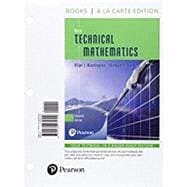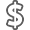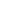# Basic Technical Mathematics, Books a la Carte Edition

• ISBN13:

• ISBN10:

## 0134435796

• Edition: 11th
• Format: Loose-leaf
• Publisher: Pearson

Note: Supplemental materials are not guaranteed with Rental or Used book purchases.

Purchase Benefits

•Free Shipping On Orders Over \$35!
Your order must be \$35 or more to qualify for free economy shipping. Bulk sales, PO's, Marketplace items, eBooks and apparel do not qualify for this offer.
•Get Rewarded for Ordering Your Textbooks! Enroll Now
•We Buy This Book Back!
In-Store Credit: \$21.00
Check/Direct Deposit: \$20.00
PayPal: \$20.00
List Price: \$173.32 Save up to \$112.83
• Rent Book \$156.42Free Shipping

TERM
PRICE
DUE
IN STOCK USUALLY SHIPS IN 24 HOURS.
*This item is part of an exclusive publisher rental program and requires an additional convenience fee. This fee will be reflected in the shopping cart.

### Supplemental Materials

What is included with this book?

• The New copy of this book will include any supplemental materials advertised. Please check the title of the book to determine if it should include any access cards, study guides, lab manuals, CDs, etc.
• The Rental and eBook copies of this book are not guaranteed to include any supplemental materials. Typically, only the book itself is included. This is true even if the title states it includes any access cards, study guides, lab manuals, CDs, etc.

### Summary

NOTE: This edition features the same content as the traditional text in a convenient, three-hole-punched, loose-leaf version. Books a la Carte also offer a great value; this format costs significantly less than a new textbook. Before purchasing, check with your instructor or review your course syllabus to ensure that you select the correct ISBN. For Books a la Carte editions that include MyLab™ or Mastering™, several versions may exist for each title — including customized versions for individual schools — and registrations are not transferable. In addition, you may need a Course ID, provided by your instructor, to register for and use MyLab or Mastering products.

For courses in technical and pre-engineering technical programs or other programs for which coverage of basic mathematics is required.

The best-seller in technical mathematics gets an “Oh, wow!” update

The 11th Edition of  Basic Technical Mathematics  is a bold revision of this classic best-seller. The text now sports an engaging full-color design, and new co-author Rich Evans has introduced a wealth of relevant applications and improvements, many based on user feedback. The text is supported by an all-new online graphing calculator manual, accessible at point-of-use via short URLs. The MyLab™ Math course features hundreds of new algorithmic exercises, tutorial videos, and PowerPoint slides.  The text continues to feature a vast number of applications from technical and pre-engineering fields—including computer design, electronics, solar energy, lasers fiber optics, and the environment—and aims to develop students’ understanding of mathematical methods without simply providing a collection of formulas. The authors start the text by establishing a solid background in algebra and trigonometry, recognizing the importance of these topics for success in solving applied problems.

Also available with MyLab Math

MyLab™ Math is an online homework, tutorial, and assessment program designed to work with this text to engage students and improve results. Within its structured environment, students practice what they learn, test their understanding, and pursue a personalized study plan that helps them absorb course material and understand difficult concepts. The MyLab Math course features hundreds of new algorithmic exercises, tutorial videos, and PowerPoint slides.

Note: You are purchasing a standalone product; MyLab Math does not come packaged with this content. If you would like to purchase both the physical text and MyLab Math, search for:

0134467086 / 9780134467085 Basic Technical Mathematics, Books a la Carte Edition plus MyLab Math with Pearson eText -- Access Card Package

Package consists of:

• 0134435796 / 9780134435794 Basic Technical Mathematics, Books a la Carte Edition
• 0321262522 / 9780321262523 MyLab Math -- Valuepack Access Card

1 Basic Algebraic Operations

1.1 Numbers

1.2 Fundamental Operations of Algebra

1.3 Calculators and Approximate Numbers

1.4 Exponents and Unit Conversions

1.5 Scientific Notation

1.7 Addition and Subtraction of Algebraic Expressions

1.8 Multiplication of Algebraic Expressions

1.9 Division of Algebraic Expressions

1.10 Solving Equations

1.11 Formulas and Literal Equations

1.12 Applied Word Problems

2 Geometry

2.1 Lines and Angles

2.2 Triangles

2.4 Circles

2.5 Measurement of Irregular Areas

2.6 Solid Geometric Figures

3 Functions and Graphs

3.1 Introduction to Functions

3.3 Rectangular Coordinates

3.4 The Graph of a Function

3.5 Graphs on the Graphing Calculator

3.6 Graphs of Functions Defined by Tables of Data

4 The Trigonometric Functions

4.1 Angles

4.2 Defining the Trigonometric Functions

4.3 Values of the Trigonometric Functions

4.4 The Right Triangle

4.5 Applications of Right Triangles

5 Systems of Linear Equations Determinants

5.1 Linear Equations and Graphs of Linear Functions

5.2 Systems of Equations and Graphical Solutions

5.3 Solving Systems of Two Linear Equations in Two Unknowns Algebraically

5.4 Solving Systems of Two Linear Equations in Two Unknowns by Determinants

5.5 Solving Systems of Three Linear Equations in Three Unknowns Algebraically

5.6 Solving Systems of Three Linear Equations in Three Unknowns by Determinants

6 Factoring and Fractions

6.1 Factoring: Greatest Common Factor and Difference of Squares

6.2 Factoring Trinomials

6.3 The Sum and Difference of Cubes

6.4 Equivalent Fractions

6.5 Multiplication and Division of Fractions

6.6 Addition and Subtraction of Fractions

6.7 Equations Involving Fractions

7.1 Quadratic Equations; Solution by Factoring

7.2 Completing the Square

7.4 The Graph of the Quadratic Function

8 Trigonometric Functions of Any Angle

8.1 Signs of the Trigonometric Functions

8.2 Trigonometric Functions of Any Angle

9 Vectors and Oblique Triangles

9.1 Introduction to Vectors

9.2 Components of Vectors

9.4 Applications of Vectors

9.5 Oblique Triangles, the Law of Sines

9.6 The Law of Cosines

10 Graphs of the Trigonometric Functions

10.1 Graphs of sin and cos x

10.2 Graphs of sin bx and cos bx

10.3 Graphs of sin (bx c) and cos (bx c)

10.4 Graphs of = tan x, y = cot x, y = sec x, y = csc x

10.5 Applications of the Trigonometric Graphs

10.6 Composite Trigonometric Curves

11.1 Simplifying Expressions with Integer Exponents

11.2 Fractional Exponents

11.5 Multiplication and Division of Radicals

12 Complex Numbers

12.1 Basic Definitions

12.2 Basic Operations with Complex Numbers

12.3 Graphical Representation of Complex Numbers

12.4 Polar Form of a Complex Number

12.5 Exponential Form of a Complex Number

12.6 Products, Quotients, Powers, and Roots of Complex Numbers

12.7 An Application to Alternating-current (ac) Circuits

13 Exponential and Logarithmic Functions

13.1 Exponential Functions

13.2 Logarithmic Functions

13.3 Properties of Logarithms

13.4 Logarithms to the Base 10

13.5 Natural Logarithms

13.6 Exponential and Logarithmic Equations

13.7 Graphs on Logarithmic and Semilogarithmic Paper

14 Additional Types of Equations and Systems of Equations

14.1 Graphical Solution of Systems of Equations

14.2 Algebraic Solution of Systems of Equations

15 Equations of Higher Degree

15.1 The Remainder and Factor Theorems; Synthetic Division

15.2 The Roots of an Equation

15.3 Rational and Irrational Roots

16 Matrices; Systems of Linear Equations

16.1 Matrices: Definitions and Basic Operations

16.2 Multiplication of Matrices

16.3 Finding the Inverse of a Matrix

16.4 Matrices and Linear Equations

16.5 Gaussian Elimination

16.6 Higher-order Determinants

17 Inequalities

17.1 Properties of Inequalities

17.2 Solving Linear Inequalities

17.3 Solving Nonlinear Inequalities

17.4 Inequalities Involving Absolute Values

17.5 Graphical Solution of Inequalities with Two Variables

17.6 Linear Programming

18 Variation

18.1 Ratio and Proportion

18.2 Variation

19 Sequences and the Binomial Theorem

19.1 Arithmetic Sequences

19.2 Geometric Sequences

19.3 Infinite Geometric Series

19.4 The Binomial Theorem

20.1 Fundamental Trigonometric Identities

20.2 The Sum and Difference Formulas

20.3 Double-Angle Formulas

20.4 Half-Angle Formulas

20.5 Solving Trigonometric Equations

20.6 The Inverse Trigonometric Functions

21 Plane Analytic Geometry

21.1 Basic Definitions

21.2 The Straight Line

21.3 The Circle

21.4 The Parabola

21.5 The Ellipse

21.6 The Hyperbola

21.7 Translation of Axes

21.8 The Second-degree Equation

21.9 Rotation of Axes

21.10 Polar Coordinates

21.11 Curves in Polar Coordinates

22 Introduction to Statistics

22.1 Graphical Displays of Data

22.2 Measures of Central Tendency

22.3 Standard Deviation

22.4 Normal Distributions

22.5 Statistical Process Control

22.6 Linear Regression

22.7 Nonlinear Regression

Appendix A Solving Word Problems

Appendix B Units of Measurement